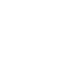# SAT & ACT Question of the Day: Jan. 19, 2014

If you are reading this in an email you received from me, do not click the link to sat.collegeboard.org below. Use the link to my website that is farther down on the email.

http://sat.collegeboard.org/practice/sat-question-of-the-day?questionId=20140119&oq=1 (This link takes you to today’s question. If you use my archive, you will see the question related to my SAT explanation for that date.)

The answer is C.  When you see equations on the SAT or ACT with radicals in them, the first thing you must do is get rid of the radicals.  To do so, simply square the radical(s) and be sure to keep the equation balanced.  In other words, if there’s a radical on one side of the equation, be sure you square both sides.  In today’s question you just square both sides by removing the radicals from both sides and end up with x-a=x+b.  When you subtract x from both sides of the equation, you are left with -a=+b which gives you a+b=0, Answer C.

That’s the algebra teacher approach and it works just fine for  most students but what if you have trouble with algebra?  Then you should use my strategy “change the algebra to arithmetic.”  Because the radicals on each side are equal, let’s just substitute a real number for the amount under the radicals on each side of the equation.  If you use a perfect square, it is going to be much easier.  I used 9.  So, now the equation is √9=√9.  That means x-a is 9 and x+b is 9.  Make up a number for x.  How about 11?  Then you know that 11-(+2) is 9 for the left side of the equation and x+b is 11+(-2) is 9 for the right side.  That means a has to be +2 and b has to be -2.  Plugging those values into the answers for a and b gives you Answer C: +2+(-2)=0.

My point is that there’s always going to be more than one way to do math problems.  You need to figure out which strategy is going to work best for you.  You have to practice in order determine that.  I recommend that you take a look at the tables in Demystifying the SAT & ACT that list the questions in the Official SAT Study Guide and Real ACT Prep Guide by math topic.  That will make finding practice questions by the topics that you find most challenging very easy.  As a bonus you’ll find the questions are also coded by difficulty level.  Using the table will allow you to easily find the questions that are going to be most helpful for raising your math score because they are organized by math topic and difficulty level.

Let’s see what the ACT folks have for us today.

The answer is B.  Use PICK.  Roman Numeral I is not Insertable because it disagrees with lines 24-5.  Roman Numeral III also disagrees with line 37; so, it isn’t Insertable either.  That leaves II which is supported by lines 36-7.

The I in PICK stand for Insertable.  Be sure you practice and use it as it is described in Video #3 and on my free website.  It is a very powerful and efficient tool for “PICKing” the best answer.

Enjoy your day.  I hope your teams win their NFL playoff games this afternoon.  I’m planning on drinking some hot chocolate and trying to stay warm while I watch the games.

The SAT & ACT Wizard